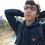# Help: Need resources on Number Theory (Kinda)

Hey,

After being a while on Brilliant, I realize a lot of the problems in the number theory and combinatorics sections contain math which I do not know.

I've always glimpsed at books or lectures which include the traditional mathematical symbols (For all, There exists, etc.) where proofs are formulated.

I'd like to know what the area of mathematics that deals with an introduction to proof and using all those symbols in number theory and combinatorics, with some traditional and theoretical mathematics.

I have a math exercise book in which a lot of the problems say "Prove..." and I have no clue what do in these cases because I certainly have know knowledge in this vast area of mathematics.

Can anyone please give me resources (books, lectures, videos, notes) such that I can try to get an introduction over the summer.

ThanksNote by Hussein Hijazi
6 years, 5 months ago

This discussion board is a place to discuss our Daily Challenges and the math and science related to those challenges. Explanations are more than just a solution — they should explain the steps and thinking strategies that you used to obtain the solution. Comments should further the discussion of math and science.

When posting on Brilliant:

• Use the emojis to react to an explanation, whether you're congratulating a job well done , or just really confused .
• Ask specific questions about the challenge or the steps in somebody's explanation. Well-posed questions can add a lot to the discussion, but posting "I don't understand!" doesn't help anyone.
• Try to contribute something new to the discussion, whether it is an extension, generalization or other idea related to the challenge.

MarkdownAppears as
*italics* or _italics_ italics
**bold** or __bold__ bold
- bulleted- list
• bulleted
• list
1. numbered2. list
1. numbered
2. list
Note: you must add a full line of space before and after lists for them to show up correctly
paragraph 1paragraph 2

paragraph 1

paragraph 2

[example link](https://brilliant.org)example link
> This is a quote
This is a quote
    # I indented these lines
# 4 spaces, and now they show
# up as a code block.

print "hello world"
# I indented these lines
# 4 spaces, and now they show
# up as a code block.

print "hello world"
MathAppears as
Remember to wrap math in $$ ... $$ or $ ... $ to ensure proper formatting.
2 \times 3 $2 \times 3$
2^{34} $2^{34}$
a_{i-1} $a_{i-1}$
\frac{2}{3} $\frac{2}{3}$
\sqrt{2} $\sqrt{2}$
\sum_{i=1}^3 $\sum_{i=1}^3$
\sin \theta $\sin \theta$
\boxed{123} $\boxed{123}$

Sort by:

This packet is an introduction to common proof arguments, and symbols.

This book contains 50 problems in combinatorics that go from the very easy to the unsolved. All of the solved problems contain detailed solutions. It is a fun way to learn and you can try for yourself before learning from the solutions.

This series of videos is from the "Math for Computer Science" class at MIT and it goes through proofs, basic number theory, as well as combinatorics topics like graphs.

Staff - 6 years, 4 months ago

Alright thanks! Good to hear about the MIT OCW because thats what I've been following for a while right now :) Let the proofs begin!

- 6 years, 4 months ago

- 6 years, 4 months ago